What is the formula of ammonium carbonate?Concept:

A chemical formula gives information about the element and their corresponding proportion in the compound.

Steps to write the chemical formula of a compound are as follows:

1. Write the letter symbol of cation and anion.

2. Identify the charge on cation and anion. Generally, group 1 elements have +1 charge, group 2 elements have  -2 charge, Group 16 elements have -2 charge, group 17 elements have  -1 charge.

3. Balance the total positive and negative charge. To balance the charges write the charge on anion as a subscript for cation and the charge on cation as a subscript for anion.

Solution:

Step:1 The ammonium ion is a polyatomic ion and the charge carried by ammonium ion is +1. Carbonate ion is also a polyatomic ion and the charge carried by carbonate ion is -2.

The letter symbol of ammonium ion is,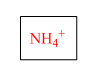The letter symbol of carbonate ion is,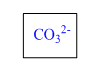Step: 2 To maintain the electrical neutrality of the compound the positive and negative charges must be equal. To balance the net charge two ammonium ions must be associated with one carbonate ion.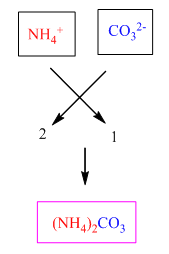Therefore the chemical formula for the compound ammonium carbonate is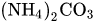.

Similar problems:

Question:

1. What is the formula of potassium nitride?

Solution:

The letter symbol of potassium is,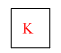The letter symbol of nitrogen is,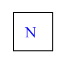Potassium is a group 1 element and thus the charge on potassium ion is +1.

Nitrogen gains 3 electrons and form nitride ion. The charge on nitride ion is -3.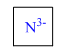To maintain the electrical neutrality of the compound the positive and negative charges must be equal. To balance the net charge three potassium ions must be associated with one nitride ion.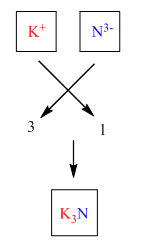Therefore the chemical formula for the compound potassium nitride is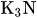.

Question:

2. What is the formula for calcium fluoride?

Solution:

The letter symbol of calcium is,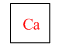The letter symbol of fluorine is,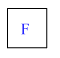Calcium is a group 2 element and thus the charge on calcium is +1.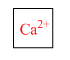Fluorine, on the other hand, is group 17 element and thus the charge on chlorine is -1.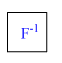To maintain the electrical neutrality of the compound the positive and negative charges must be equal. To balance the net charge two fluorine atoms must be associated with one atom of calcium.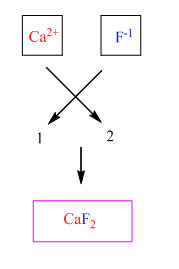Therefore the chemical formula for the compound magnesium chloride is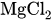.

Need homework help now?

tutors online

Related Questions
2. (11 marks) a) At 400 °C, ammonium iodide decomposes in the following endothermic reaction: NH41(s) + NH3(g) + HI(g) Kp = 0.215 at 400 °C. Write the equilibrium expression for this reaction. 15.0 g of NH4I was placed in an empty 3.0 L flask and heated to 400 °C. What is the partial pressure of NH3 in the flask once the reaction has reached equilibrium? How would the following changes to the equilibrium mixture affect the number of moles of NH3 in the flask and the value of K, once equilibrium is re-established? (Use increase, decrease or stay the same.) Change Effect on moles of NH3 Ke value Increase temperature Increase volume of flask Remove half of HI gas
Notes
Me

OR

Don't have an account?

Join OneClass

Access over 10 million pages of study
documents for 1.3 million courses.

Join to view

OR

By registering, I agree to the Terms and Privacy Policies
Just a few more details

So we can recommend you notes for your school.